Home | | Transforms and Partial Differential Equations | Applications of Partial Differential Equations

# Applications of Partial Differential Equations

1 Introduction 2 Solution of Wave Equation 3 Solution of The Heat Equation 4 Solution of Laplace Equations(Two dimensional heat equation)

APPLICATIONS OF PARTIAL DIFFERENTIAL EQUATIONS

1 INTRODUCTION

2 SOLUTION OF WAVE EQUATION

3 SOLUTION OF THE HEAT EQUATION

4 SOLUTION OF LAPLACE EQUATIONS

1 INTRODUCTION

In Science and Engineering problems, we always seek a solution of the differential equation which satisfies some specified conditions known as the boundary conditions. The differential equation together with the boundary conditions constitutes a boundary value problem. In the case of ordinary differential equations, we may first find the general solution and then determine the arbitrary constants from the initial values. But the same method is not applicable to partial differential equations because the general solution contains arbitrary constants or arbitrary functions. Hence it is difficult to adjust these constants and functions so as to satisfy the given boundary conditions. Fortunately, most of the boundary value problems involving linear partial differential equations can be solved by a simple method known as the method of separation of variables which furnishes particular solutions of the given differential equation directly and then these solutions can be suitably combined to give the solution of the physical problems.

2Solution of the wave equation

The wave equation isLet y = X(x) . T(t) be the solution of (1), where â€žXâ€ź is a function of â€žxâ€ź only and â€žTâ€ź is a function of â€žtâ€ź only.Of these three solutions, we have to select that particular solution which suits the physical nature of the problem and the given boundary conditions. Since we are dealing with problems on vibrations of strings, â€žyâ€ź must be a periodic function of â€žxâ€ź and â€žtâ€ź.Now the left side of (2) is a function of â€žxâ€ź only and the right side is a function of â€žtâ€ź only.

Since â€žxâ€ź and â€žtâ€ź are independent variables, (2) can hold good only if each side is equal to a constant.

Hence the solution must involve trigonometric terms.

Therefore, the solution given by (5),

i.e,     y = (c5 coslx  + c6 sin lx) (c7 cosalt+ c8 sin alt)

is the only suitable solution of the wave equation.

llustrative Examples.

Example 1

If a string of length â„“ is initially at rest in equilibrium position and each of its points is given the velocitySolution

The displacement y(x,t) is given by the equationThe boundary conditions are

i.           y(0,t) = 0, for t Âł0.

ii.    y(â„“,t)  Âł0.=   0,   for     t

y(x,0) = 0, for 0 ÂŁÂŁâ„“.Since the vibration of a string is periodic, therefore, the solution of (1) is of the form

y(x,t) = (Acoslx + Bsinlx)(Ccoslat + Dsinlat) ------------(2)

Using (i) in (2) , we get

0 = A(Ccoslat + Dsinlat) , for all t Âł0.

Therefore,            A = 0

Hence equation (2) becomes

y(x,t) = B sinlx(Ccoslat + Dsinlat) ------------ (3)

Using (ii) in (3), we get

0 = Bsinlâ„“   (Ccoslat+Dsinlat), for all  t Âł0, which gives lâ„“ = np

Hence,         l= np / l , n being an integer.Using condition (iv) in the above equation, we getExample 2

A tightly stretched string with fixed end points x = 0 & x = â„“ is initially at rest in its equilibrium position . If it is set vibrating by giving to each of its points a  velocity

Â¶y/Â¶t    = kx(â„“-x) at t = 0. Find the displacement y(x,t).

Solution

The displacement y(x,t) is given by the equationThe boundary conditions areExample 3

A tightly stretched string with fixed end points x = 0 & x = â„“ is initially in a position given by y(x,0) = y0sin3(px/â„“). If it is released from this position, find the displacement y at any time and at any distance from the end x = 0 .

Solution

The displacement y(x,t) is given by the equationThe boundary conditions are

(i)     y(0,t) = 0,  "Âł0.

(ii)                                     y("tâ„“Âł,t)0.     =   0,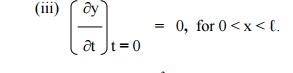(iv)  y(x,0) = y0 sin3((px/â„“),   for   0   <   x   <   â„“.

The suitable solution of (1) is given by

y(x,t) = (Acoslx + Bsinlx)(Ccoslat + Dsinlat) ------------(2)

Using (i) and (ii) in (2) , we get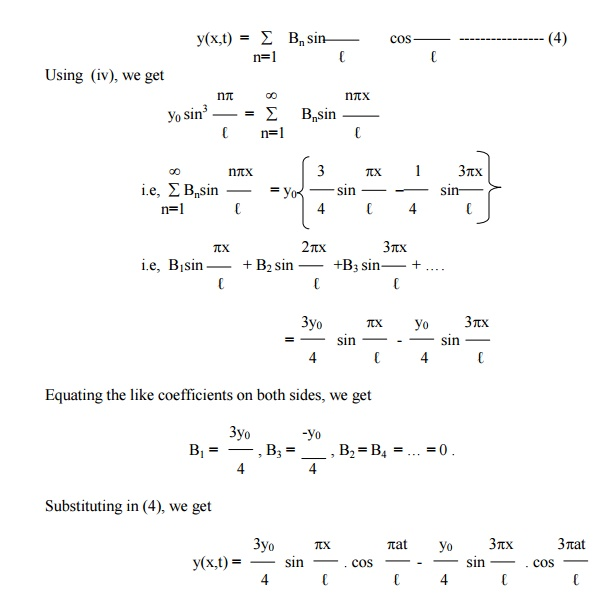Example 4

A string is stretched & fastened to two points x = 0 and x = â„“ apart. Motion is started by displacing the string into the form y(x,0) = k(â„“x-x2) from which it is released at time t = 0. Find the displacement y(x,t).

Solution

The displacement y(x,t) is given by the equationThe boundary conditions are

(i)     y(0,t) = 0,  "Âł0.

(ii)   y(â„“,t) "Âł=0.  0,The suitable solution of (1) is given by

y(x,t) = (Acoslx + Bsinlx)(Ccoslat + Dsinlat) ------------(2)

Using    (i) and (ii) in (2) , we get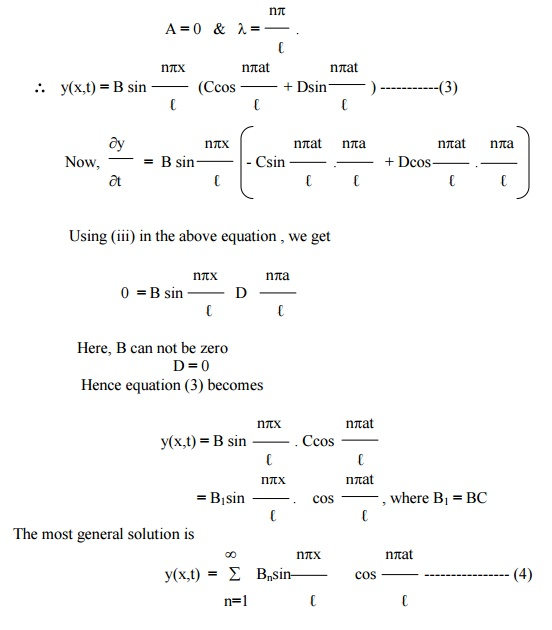Example 5

A uniform elastic string of length 2â„“ is fastened at both ends. The midpoint of the string is taken to the height â€žbâ€ź and then released from rest in  that position . Find the displacement of the string.

Solution

The displacement y(x,t) is given by the equation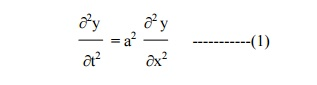The suitable solution of (1) is given by

y(x,t) = (Acoslx + Bsinlx)(Ccoslat + Dsinlat)      ------------(2)

The boundary conditions are

(i)     y(0,t) = 0,  "Âł0.

(ii)   y(â„“,t) "Âł=0.  0,[Since,   equation   of   OA   is(y- b)/(oy-b)== (x(b/-â„“)/(2â„“-â„“)x)]â„“

Using conditions (i) and (ii) in (2), we getHere B can not be zero, therefore      D = 0.

Hence equation (3) becomesExample 6

A tightly stretched string with fixed end points x = 0 & x = â„“ is initially in  the position y(x,0) = f(x). It is set vibrating by giving to each of its points a  velocity   Â¶y/Â¶t = g(x) at t = 0 . Find the displacement y(x,t) in the form of Fourier series.

Solution

The displacement y(x,t) is given by the equation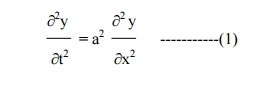The boundary conditions are

(i)     y(0,t) = 0,  "Âł0.

(ii)   y(â„“,t) "Âł=0.  0,

(iii) y(x,0) = f(x) , for 0 ÂŁÂŁâ„“.=g(x) , for 0 ÂŁÂŁâ„“. Â¶t t

The solution of equation .(1) is given by

y(x,t) = (Acoslx + Bsinlx)(Ccoslat + Dsinlat) ------------(2)

where A, B, C, D are constants.

Applying conditions (i) and (ii) in (2), we have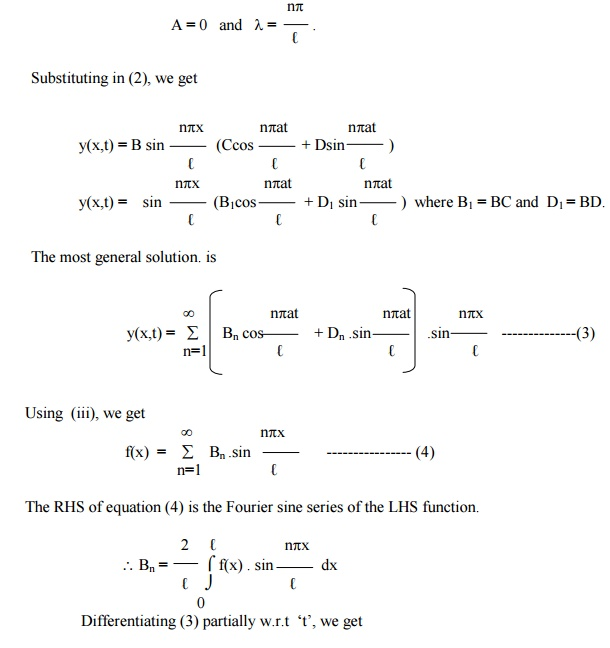Substituting the values of Bn and Dn in (3), we get the required solution of the given equation.

Exercises

(1) Find the solution of the equation of a vibrating string of   length   'â„“',   satisfying the conditions

y(0,t) = y(â„“,t) = 0 and y = f(x), Â¶y/ Â¶t = 0 at t = 0.

(2) A taut string of length 20 cms. fastened at both ends is displaced from its position of equilibrium, by imparting to each of its points an initial velocity given by

v = x      in 0 ÂŁÂŁ10

= 20 -x  in 10 ÂŁÂŁ20,

â€žxâ€ź being the distance from one end. Determine the displacement at any subsequent time.

(3) Find the solution of the wave equation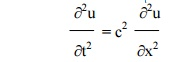corresponding to the triangular initial deflection f(x ) = (2k/ â„“)   x   where 0<x<â„“/2

= (2k/ â„“) â€“x)(â„“-x)       where â„“/2<x<â„“,

and initial velocity zero.

(4) A tightly stretched string with fixed end points x = 0 and x = â„“ is initially at rest in its equilibrium position. If it is set vibrating by giving to each of its points a velocity Â¶y/ Â¶= f(x)

at t = 0. Find the displacement y(x,t).

(5) Solve the following boundary value problem of vibration of string

i.           y(0,t) = 0

ii.           y(â„“,t)   =   0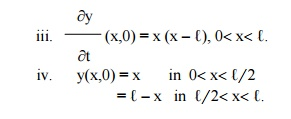(6) A tightly stretched string with fixed end points x = 0 and x = â„“ is initially in a position given by y(x,0) = k( sin(px/ â„“) â€“ sin( 2px/ â„“)). If it is released from rest, find the displacement of â€žyâ€ź at any distance â€žxâ€ź from one end at any time "tâ€ź.

3 Solution of the heat equation

The heat equation is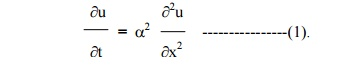Let u = X(x) . T(t) be the solution of (1), where â€žXâ€ź is a function of â€žxâ€ź alone and â€žTâ€ź is a function of â€žtâ€ź alone.

Substituting these in (1), we getNow the left side of (2) is a function of â€žxâ€ź alone and the right side is a function of â€žtâ€ź  alone. Since â€žxâ€ź and â€žtâ€ź are independent variables, (2) can be true only if each side is  equal to a constant.Hence, we get Xâ€˛â€˛ - kX = 0 and Tâ€˛ -a2kT=0.-------------- (3).

Solving equations (3), we get

(i)                                when   â€žkâ€ź, is say   positive   and   k   = l2

X = c1 elx  + c2  e - lx(iii)     when   â€žkâ€ź   is   zero.

X = c7 x + c8

T = c9

Thus the various possible solutions of the heat equation (1) areOf these three solutions, we have to choose that solution which suits the physical nature of the problem and the given boundary conditions. As we are dealing with problems on heat flow, u(x,t) must be a transient solution such that â€žuâ€ź is to decrease with the increase of time â€žtâ€ź.

Therefore, the solution given by (5),is the only suitable solution of the heat equation.

Illustrative Examples

Example 7

A rod â€žâ„“â€ź cm with insulated lateral surface is initially at temperature f(x) at an inner point of distance x cm from one end. If both the ends are kept at zero temperature, find the temperature at any point of the rod at any subsequent time.The boundary conditions are

(i)u (0,t) = 0,      " t   â‰Ą   0

(ii)   u   (â„“,t) "t > 0 =   0,

(iii)  u   (x,0)   =   f   (x),   0   <   x   <   â„“

The solution of equation (1) is given byneglecting radiation. Find an expression for u, if the ends of the bar are maintained at zero temperature and if, initially, the temperature is T at the centre of the bar and falls uniformly to zero at its ends.Let u be the temperature at P, at a distance x from the end A at time t.

The temperature function u (x,t) is given by the equation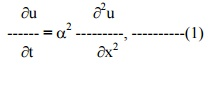The boundary conditions are

(i)                u (0,t)  = 0, "> 0.

(ii)             u   (â„“=0,,t)"> 0.The solution of (1) is of the form

Applying conditions (i) and (ii) in (2), we getSteady - state conditions and zero boundary conditions Example 9

A rod of length â€žâ„“â€ź has its ends A and B kept at 0Â°C and 100Â°C until steady state   conditions prevails. If the temperature at B is reduced suddenly to 0Â°C and kept so while that of A is maintained, find the temperature u(x,t) at a distance x from A and at time â€žtâ€ź.

The heat-equation is given byPrior to the temperature change at the end B, when t = 0, the heat flow was independent of time (steady state condition).

When the temperature u depends only on x, equation(1) reduces to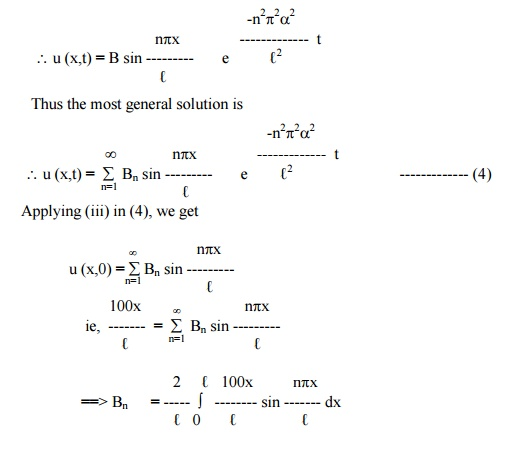Example 10

A rod, 30 c.m long, has its ends A and B kept at 20Â°C and 80Â°C respectively, until steady state conditions prevail. The temperature at each end is then suddenly reduced to 0Â°C and kept so. Find the resulting temperature function u (x,t) taking x = 0 at A.

The one dimensional heat flow equation is given byThe initial conditions, in steady â€“state, are

u = 20, when        x = 0

u = 80, when        x = 30

Therefore, (3) gives b = 20, a = 2.

u (x) = 2x + 20     ------------- (4)

Hence the boundary conditions are

(i)      u (0,t) = 0,  " t > 0

(ii)     u (30,t) = 0, " t > 0

(iii)    u (x,0)         = 2x + 20, for 0 < x < 30

The solution of equation (1) is given byApplying conditions (i) and (ii), we getSteadyâ€“state conditions and nonâ€“zero boundary conditions

Example 11

The ends A and B of a rod 30cm. long have their temperatures kept at 20Â°C and 80Â°C, until steadyâ€“state conditions prevail. The temperature of the end B is suddenly reduced to 60Â°C and kept so while the end A is raised to 40Â°C. Find the temperature distribution in the rod after time t.

Let the equation for the heat- flow beThe initial conditions, in steadyâ€“state, are

u = 20,        when x =  0

u = 80,        when x = 30

From (2), b = 20  & a = 2.

Thus the temperature function in steadyâ€“state is

u (x) = 2x + 20--------------       (3)

Hence the boundary conditions in the transientâ€“state are

(i)      u (0,t) = 40, " t > 0

(ii)     u (30,t) = 60,        " t > 0

(iii)    u (x,0) = 2x + 20, for 0 < x < 30

we break up the required funciton u (x,t) into two parts and write

u (x,t) = us (x) + ut (x,t)--------------- (4)

where us (x) is a solution of (1), involving x only and satisfying the boundary condition (i) and (ii). ut (x,t) is then a function defined by (4) satisfying (1).

Thus us(x) is a steady state solution of (1) and ut(x,t) may therefore be regarded as a transient solution which decreases with increase of t.

To find us(x)

we have to solve the equationSolving, we get us(x) = ax + b           ------------- (5)

Here us(0) = 40, us(30) = 60.

Using the above conditions, we get b = 40, a = 2/3.To find ut(x,t)

ut ( x,t) = u (x,t) â€“us (x)

Now putting x = 0 and x = 30 in (4), we have

ut (0,t) = u (0,t)  â€“u(0) =         40â€“40 = 0

and   ut (30,t) = u (30,t) â€“u(30) = 60â€“60 = 0

Also u(x,0) = u (x,0) â€“u(x)Hence the boundary conditions relative to the transient solution ut (x,t) are

ut (0,t)  = 0

ut (30,t) = 0  --------------(v)

and ut (x,0) = (4/3) x â€“20 ------------- (vi)Exercises

(1) Solve Â¶u/ Â¶t = a2 (Â¶2/ Â¶x2) subject to the boundary conditions u(0,t) = 0, u(l,t) = 0, u(x,0) = x, 0<x<l.

(2)     Find the solution to the equation  Â¶u/ Â¶t = a2 (Â¶2/ Â¶x2) that satisfies the conditions

i.           u(0,t) = 0,

ii.           u(l,t) = 0, ">0,

iii.    u(x,0) = x  for 0<x<l/ 2.

=    l â€“x  for l/ 2<x<l.

(3)   Solve the equation  Â¶u/ Â¶t = a2 (Â¶2/ Â¶x2) subject to the boundary conditions

i.           u(0,t) = 0,

ii.           u(l,t) = 0, ">0,

iii.           u(x,0) = kx(l â€“x), k >0, 0 ÂŁÂŁl.

(4) A rod of length â€žlâ€ź has its ends A and B kept at 0 o  C and 120 o   C respectively until steady state conditions prevail. If the temperature at Bis reduced to 0 o  C and kept so while that of A is maintained, find the temperature distribution in the rod.

(5) A rod of length â€žlâ€ź has its ends A and B kept at 0o C and 120 o  C respectively until steady state conditions prevail. If the temperature at Bis reduced to 0 o  C and kept so while 10 o  C and at the same instant that at A is suddenly raised to 50 o  C. Find the temperature distribution in the rod after time â€žtâ€ź.

(6) A rod of length â€žlâ€ź has its ends A and B kept at 0 o  C and 100 o  C respectively until steady state conditions prevail. If the temperature of A is suddenly raised to 50 o  C and that of B to 150 o  C, find the temperature distribution at the point of the rod and at any time.

(7)   A rod of length 10 cm. has the ends A and B kept at temperatures 30o C and 100o C, respectively until the steady state conditions prevail. After some time, the temperature at A is lowered to 20o C and that of B to 40o C, and then these temperatures are maintained. Find the subsequent temperature distribution.

(8)   The two ends A and B of a rod of length 20 cm. have the temperature at 30o C and 80o C respectively until th steady state conditions prevail. Then the temperatures at the ends A and B are changed to 40o C and 60o C respectively. Find u(x,t).

(9)   A bar 100 cm. long, with insulated sides has its ends kept at 0o C and 100o C until steady state condition prevail. The two ends are then suddenly insulated and kept so. Find the temperature distribution

(10) Solve the equation  Â¶u/ Â¶t = a2 (Â¶2/ Â¶x2)   ) subject to the conditions (i) â€žuâ€ź is not infinite

as t Â®ÂĄ (ii) u = 0 for x = 0 and x = p"t (iii) u = p-x2 for t = 0 in (0, p).

4 Solution   of   Laplaceâ€™s equation  (Two dimensional heat equation)

The Laplace equation isLet u = X(x) . Y(y) be the solution of (1), where â€žXâ€ź is a function of â€žxâ€ź alone and â€žYâ€ź is  a function of â€žyâ€ź alone.Now the left side of (2) is a function of â€žxâ€ź alone and the right side is a function of â€žtâ€ź alone. Since â€žxâ€ź and â€žtâ€ź are independent variables, (2) can be true only if each side is equal to a constant.Solving equations (3), we get

(i) when â€žkâ€ź is positive and k = l2, say

X = c1 elx  + c2  e - lx

Y = c3 cosly + c4 sin ly(iii)               when   â€žkâ€ź   is   zero.

X = c9  x + c10

Y = c11 x + c12

Thus the various possible solutions of (1) areOf these three solutions, we have to choose that solution which suits the physical nature of the problem and the given boundary conditions.

Example 12

An infinitely long uniform plate is bounded by two parallel edges x = 0 & x = â„“ and an end at right angles to them. The breadth of this edge y = 0 is â„“ and this edge is maintained at a temperature f (x). All the other 3 edges are at temperature zero. Find the steady state temperature at any interior point of the plate.

Solution

Let u (x,y) be the temperature at any point x,y of the plate.

Also u (x,y) satisfies the equationUsing (7) in (5), we get the required solution.

Example 13

A rectangular plate with an insulated surface is 8 cm. wide and so long compared to its width that it may be considered as an infinite plate. If the temperature along short edge y = 0 is u(x,0) = 100 sin (px/8), 0 < x < 8, while two long edges x = 0 & x = 8 as well as the other short edges are kept at 0Â°C. Find the steady state temperature at any point of the plate.

Solution

The two dimensional heat equation is given byThe boundary conditions are

(i) u (0, y) = 0,      for 0 < y < ÂĄ

(ii) u (8, y) = 0,     for 0 < y < ÂĄ

(iii) u (x, ÂĄ) = 0,   for 0 < x < 8

(iv) u (x, 0) = 100 Sin (ÂĄx/8,) for 0 < x < 8

Using conditions (i), & (ii), we getComparing like coefficients on both sides, we get

D1 = 100, D2 = D3 = . . . . = 0

Substituting in (4), we get

u (x,y) = 100 e(-py / 8)     sin (px / 8)

Example 14

A rectangular plate with an insulated surface 10 c.m wide & so long compared to its width that it may considered as an infinite plate. If the temperature at the short edge y = 0 is given byand all the other 3 edges are kept at temperature 0Â°C. Find the steady state temperature at any point of the plate.

Solution

The temperature function u (x,y) is given by the equationThe boundary conditions are

(i) u (0, y)   = 0,             for 0 < y < ÂĄ

(ii) u (10, y) = 0,            for 0 < y < ÂĄ

(iii) u (x, ÂĄ) = 0,            for 0 < x  < 10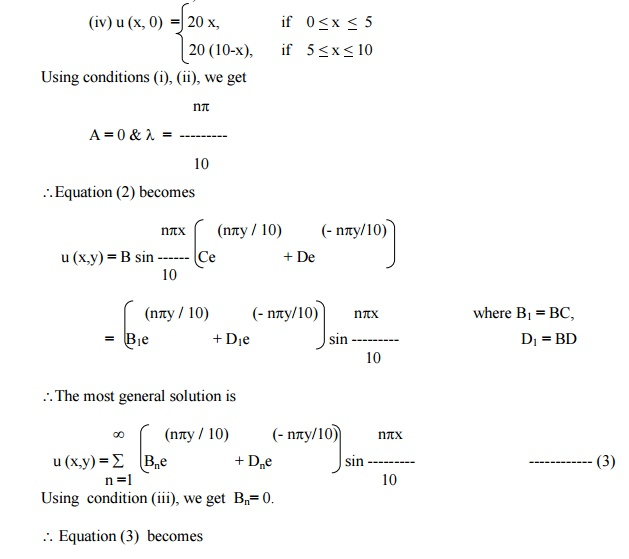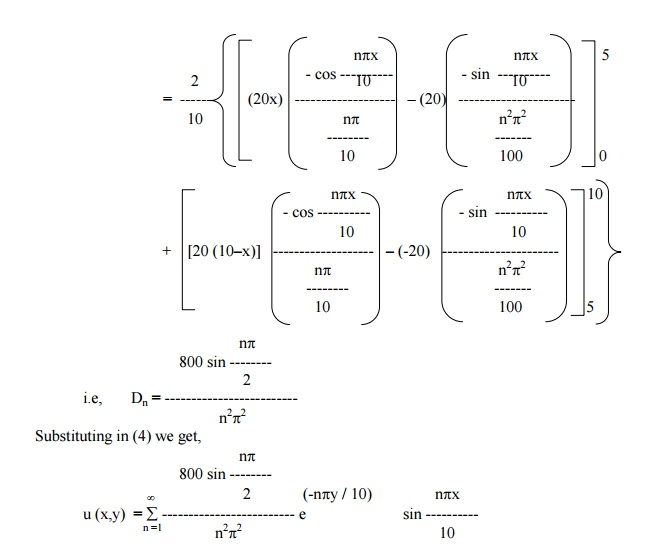Example 15

A rectangular plate is bounded by the lines x = 0, x = a, y = 0 & y = b. The edge temperatures are u (0,y) = 0, u (x,b) = 0, u (a,y) = 0 & u (x,0) = 5 sin (5px / a) + 3 sin (3px / a). Find the steady state temperature distribution at any point of the plate.

The temperature function u (x,y) satisfies the equationThe boundary conditions are

(i) u (0,y) = 0,                          for 0 < y < b

(ii) u (a,y) = 0,                         for 0 < y < b

(iii) u (x, b) = 0,    for 0 < x < a

(iv) u (x,0) = 5 sin (5px / a) + 3 sin (3px / a),       for 0 < x < a.Exercisesii.           u(a,y) = 0 for 0 <<b

iii.           u(x,b) = 0 for 0 <<a

iv.          u(x,0) = sin3(px/ a) ,0 <<a.

(2)   Find the steady temperature distribution at points in a rectangular plate with insulated faces and the edges of the plate being the lines x = 0, x = a, y = 0 and y = b. When three  of the edges are kept at temperature zero and the fourth at a fixed temperature ao C.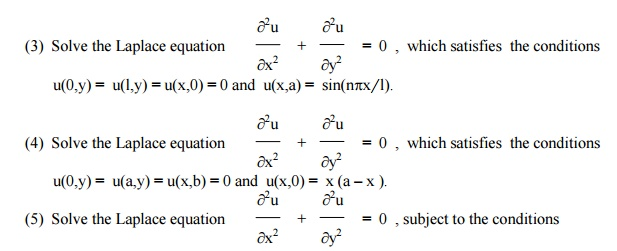i.           u(0,y) = 0, 0 ÂŁÂŁl   ii.   u(l,y) = 0, 0 ÂŁÂŁ

iii.         u(x,0) = 0, 0 ÂŁÂŁl iv.  u(x,l) = f(x), 0 ÂŁÂŁl

(6)   A square plate is bounded by the lines x = 0, y = 0, x = 20 and y = 20. Its faces are insulated.

The temperature along the upper horizontal edge is given by u(x,0) = x (20 â€“x), when 0 < x <20,

while other three edges are kept at 0o C. Find the steady state temperature in the plate.

(7) An infinite long plate is bounded plate by two parallel edges and an end at right

angles to them.The breadth is p. This end is maintained0â€źatat a c all points and the other edges are at zero temperature. Find the steady state temperature at

any point (x,y) of the plate.

(8) An infinitely long uniform plate is bounded by two parallel edges x = 0 and x = l, and

an end at right angles to them. The breadth of this edge y = 0 is â€žlâ€ź and temperature f(x). All the other three edges are at temperature zero. Find the steady state

temperature at any interior point of the plate.

(9) A rectangular plate with insulated surface is 8 cm. wide and so long compared to its width that it may be considered infinite in length without introducing an appreciable error. If the temperature along one short edge y = 0 is given by u(x,0) = 100 sin(px/ 8), 0 <<8, while the two long edges x = 0 and x = 8 as well as the other short edge are kept at 0o C, show that the steady state temperature at any point of the plane is given by u(x,y) = 100 e-py/ 8 sin px/ 8 .

(10) A rectangular plate with insulated surface is 10 cm. wide and so long compared to its width that it may be considered infinite length. If the temperature along short edge y = 0 is given

u(x,0) = 8 sin(px/ 10) when 0 <<10, while the two long edges x = 0 and x = 10 as well as the other short edge are kept at 0o C, find the steady state temperature distribution u(x,y).

Study Material, Lecturing Notes, Assignment, Reference, Wiki description explanation, brief detail
Mathematics (maths) : Applications of Partial Differential Equations : Applications of Partial Differential Equations |